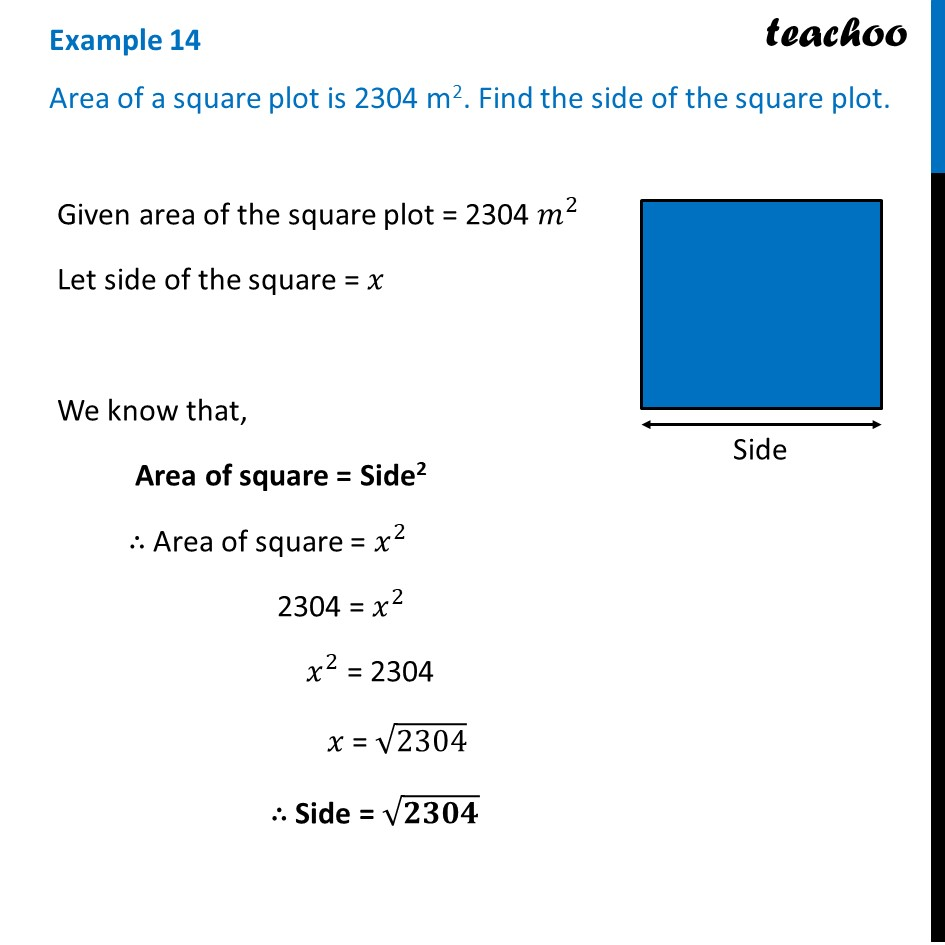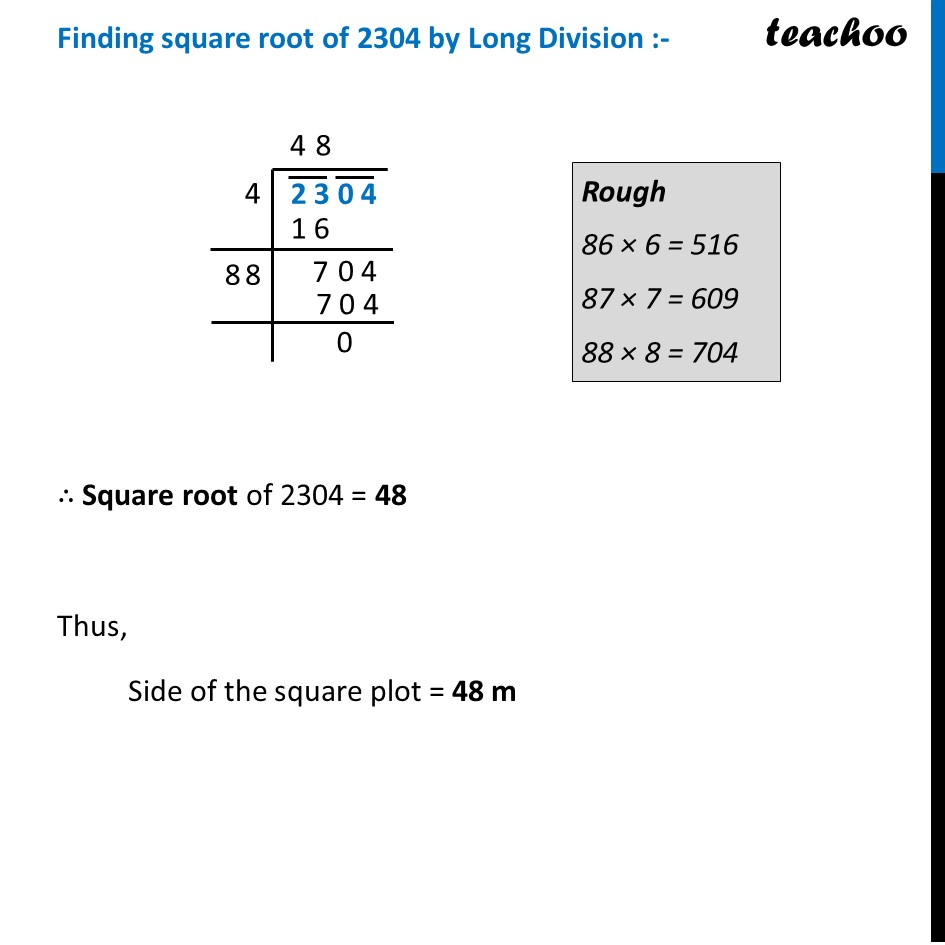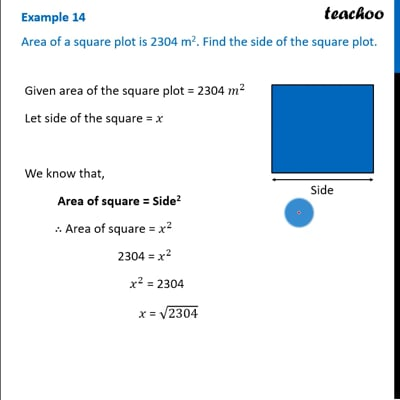Examples

Chapter 6 Class 8 Squares and Square Roots
Serial order wiseThis video is only available for Teachoo black users

### Transcript

Example 14 Area of a square plot is 2304 m2. Find the side of the square plot. Given area of the square plot = 2304 𝑚^2 Let side of the square = 𝑥 We know that, Area of square = Side2 ∴ Area of square = 𝑥^2 2304 = 𝑥^2 𝑥^2 = 2304 𝑥 = √2304 ∴ Side = √𝟐𝟑𝟎𝟒 Finding square root of 2304 by Long Division :- ∴ Square root of 2304 = 48 Thus, Side of the square plot = 48 m Rough 86 × 6 = 516 87 × 7 = 609 88 × 8 = 704